StatLect

# Discrete random variable

A random variable is said to be discrete if the set of values it can take (its support) has either a finite or an infinite but countable number of elements. Its probability distribution can be characterized through a function called probability mass function.## Definition

The following is a formal definition.

Definition A random variableis discrete if its supportis countable and there exist a function, called probability mass function of, such thatwhereis the probability thatwill take the value.

A discrete random variable is often said to have a discrete probability distribution.

## Examples

Here are some examples.

### Example 1

Letbe a random variable that can take only three values (,and), each with probability. Then,is a discrete variable. Its support isand its probability mass function is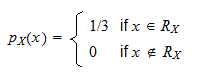So, for example, the probability thatwill be equal toisand the probability thatwill be equal toisbecausedoes not belong to the support of.

### Example 2

Letbe a random variable. Let its support be the set of natural natural numbers, that is,and its probability mass function be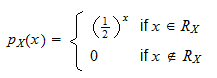Note that differently from the previous example, where the support was finite, in this example the support is infinite.

What is the probability thatwill be equal to? Sinceis a natural number, it belongs to the support ofand its probability isWhat is the probability thatwill be equal to? Sinceis not a natural number, it does not belong to the support. As a consequence, its probability is## Probability of a set of numbers

How do we compute the probability that the realization of a discrete variablewill belong to a given set of numbers?

This is accomplished by summing the values of the probability mass function over all the elements of: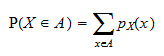Example Consider the variableintroduced in Example 2 above. Suppose we want to compute the probability thatbelongs to the setThen,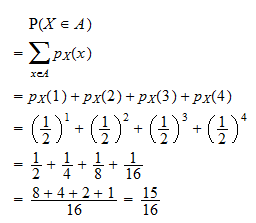## Expected value

The expected value of a discrete random variable is computed with the formulaNote that the sum is over the whole support.

Example Consider a variable having supportand probability mass function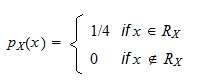Its expected value is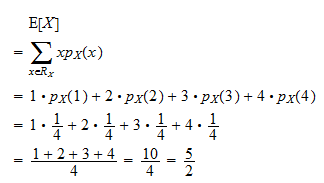## Variance

By using the definition of variance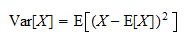and the formula for the expected value illustrated in the previous section, we can write the variance of a discrete random random variable as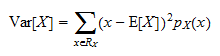Example Take the variable in the previous example. We have already calculated its expected value:Its variance is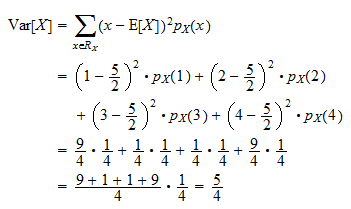## Common discrete distributions

The next table contains some examples of discrete distributions that are frequently encountered in probability theory and statistics.

Name of the discrete distribution Support Type of support
Bernoulli {0,1} Finite
Binomial {0,1,2,...,n} Finite
Poisson The set of all non-negative integer numbers Infinite but countable

## More details

You can read a thorough explanation of discrete random variables in the lecture entitled Random variables.

You can also find more details about the probability mass function in this glossary entry.

## Keep reading the glossary

Previous entry: Design matrix

Next entry: Discrete random vector

The book

Most of the learning materials found on this website are now available in a traditional textbook format.

Glossary entries
Share